# Preface

This section presents analysis of hard spring model, derived by Georg Duffing (1861–1944) in 1918.

Introduction to Linear Algebra

# Undamped Driven Hard Spring

We consider second-order differential equations of the general form $$\ddot{x} + f\left( x, \dot{x} \right) = F(t) ,$$ when F(t) is a periodic function, of which we consider three of them:
$F(t) = \Gamma\, \sin \omega t , \qquad F(t) = \Gamma\, \cos \omega t , \qquad \mbox{and} \qquad F(t) \equiv \mbox{sqw}(t) = \mbox{sign} \left( F(t) \right) ,$
where sign is the signum function. Periodic input functions are dictated by applications to spring-mass systems and electric circuits.
A solution to the differential equation $$\ddot{x} + \delta\,\dot{x} + x + \beta\,x^3 = \Gamma\,\cos (\omega t)$$ is called harmonic provided it is periodic with fundamental period P = 2π/ω. A solution to this equation is called subharmonic if it is periodic with fundamental period of an integer multiple of the forcing period P. If the fundamental period of the solution is nP where n is a positive integer greater than 1, then the solution is called a subharmonic solution of order 1/n.
Example: In this example, we investigate the behavior of solutions for the undamped Duffing equation
$\ddot{x} + x + \beta\,x^3 = F(t) ,$
where F is a periodic function
$F(t) = \Gamma \,\cos (\omega \,t ) \qquad\mbox{or} \qquad F(t) = \Gamma \,\sin (\omega \,t )$
or their discrete approximation sign(F). The main Mathematica code to do this is quite simple:
sol = NDSolve[{x''[t] + x[t] + (1/10)*(x[t])^3 == (1/5)* Sign[Sin[1.5*t]], x == 0, x' == 0}, x, {t, 0, 100}] ParametricPlot[{x[t], x'[t]} /. sol, {t, 0, 100}]
We summarize our numerical expewriments in the following tables that show how graphs depend on numerical values of parameters.
F = (1/5) sign(sin(1.5 t)) F = (1/5) sin(1.5 t) F = (1/5) sign(cos(1.5 t)) F = (1/5) cos(1.5 t)
β = 1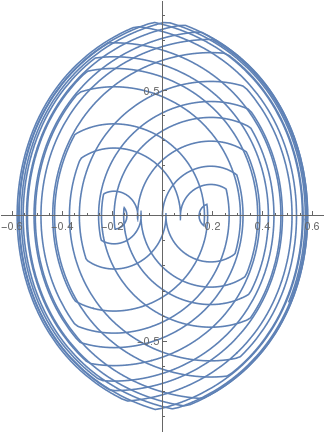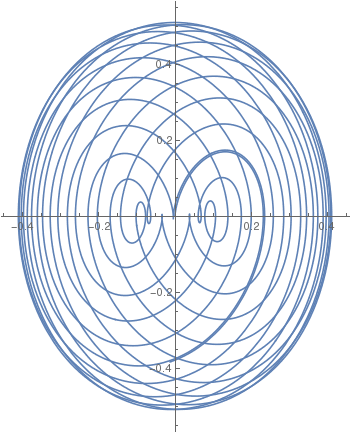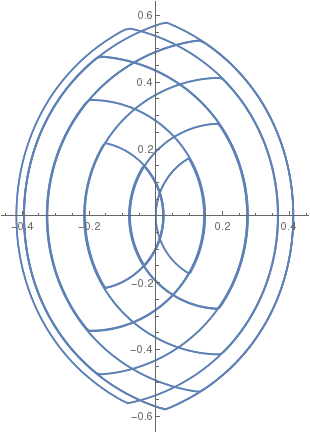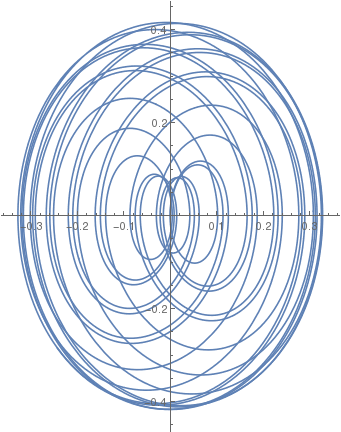β = 1/3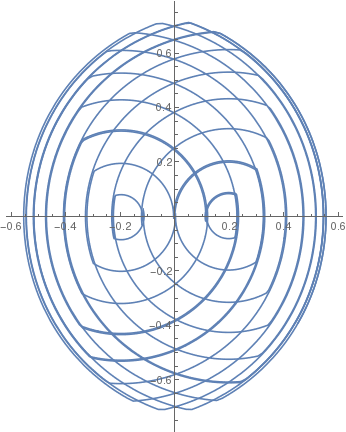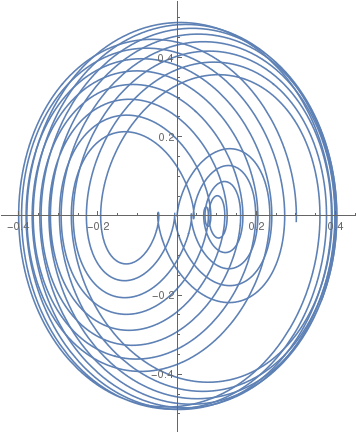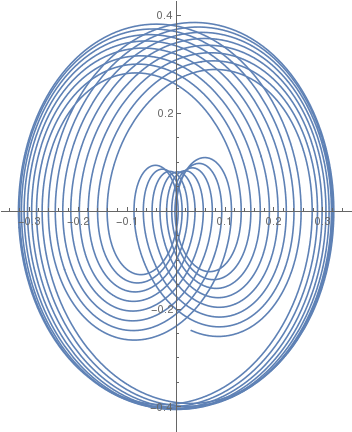β = 1/5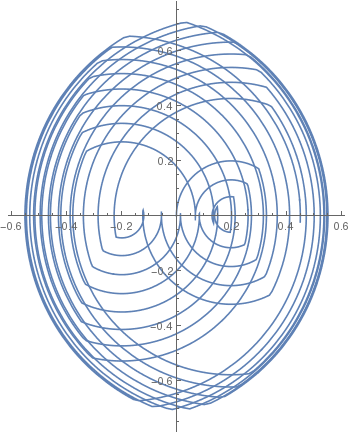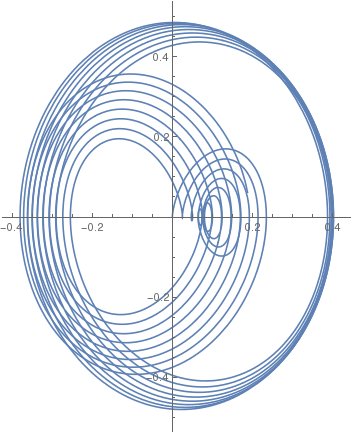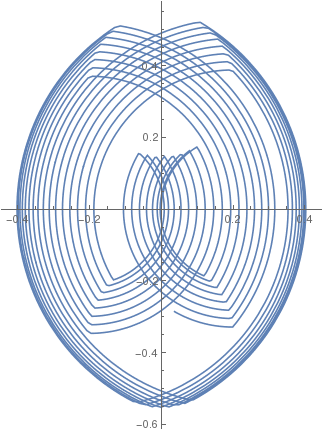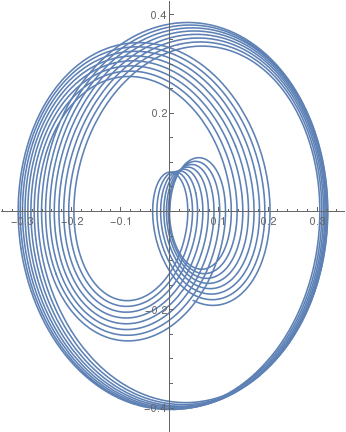β = 1/10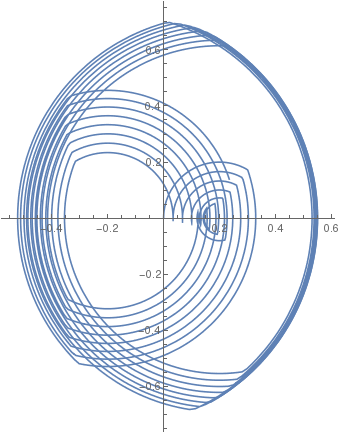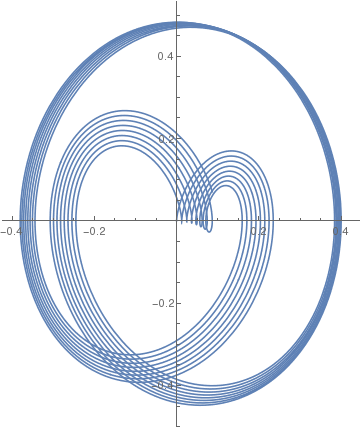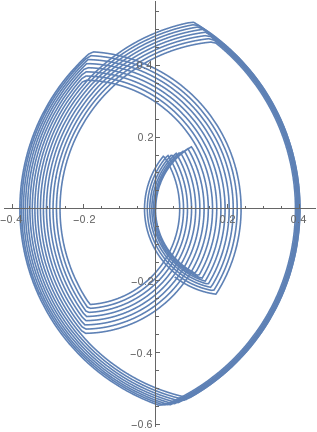Incresing frequency, we get the following graphs:
F = (1/5) sign(sin(2.0 t)) F = (1/5) sin(2.0 t) F = (1/5) sign(cos(2.0 t)) F = (1/5) cos(2.0 t)
β = 1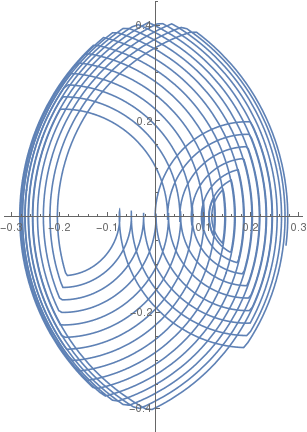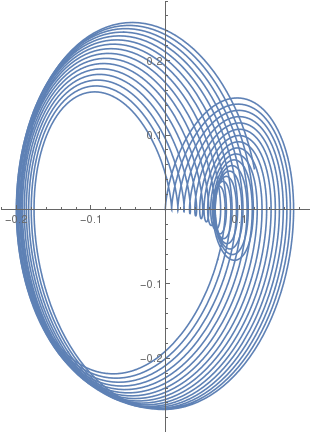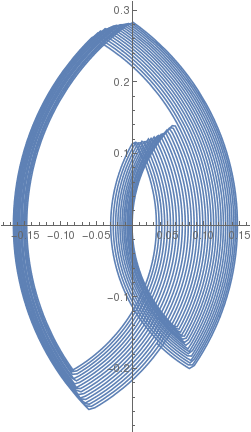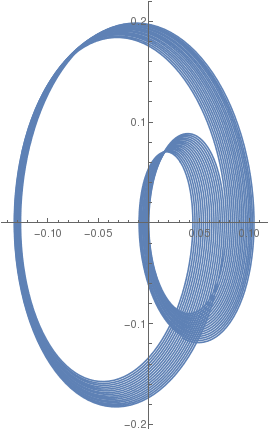β = 1/3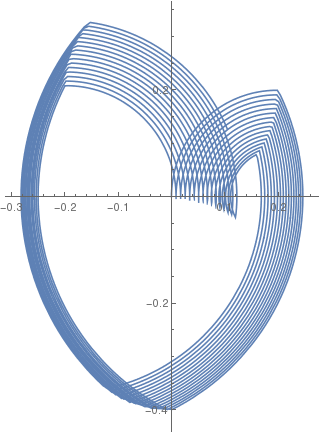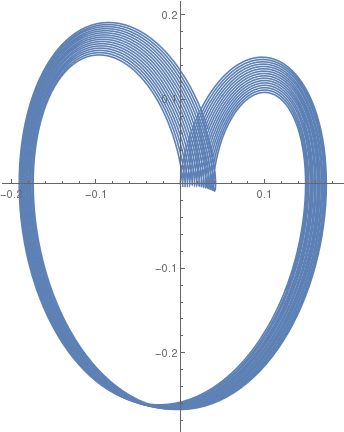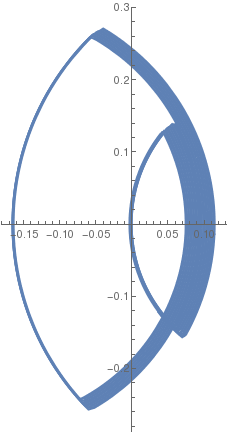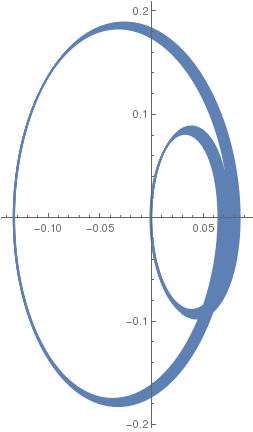β = 1/5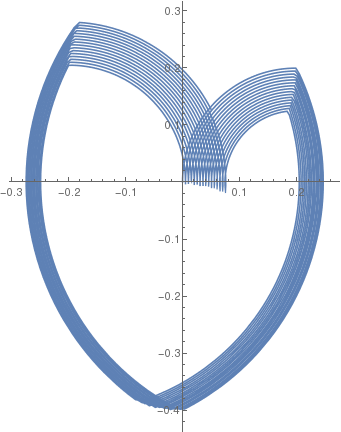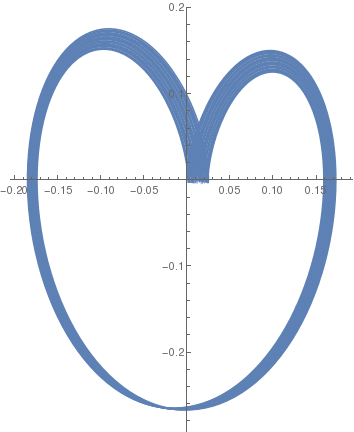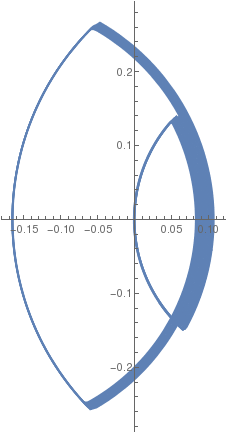β = 1/10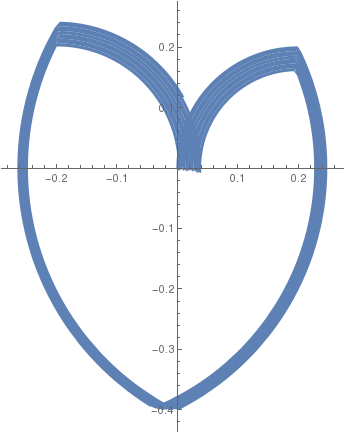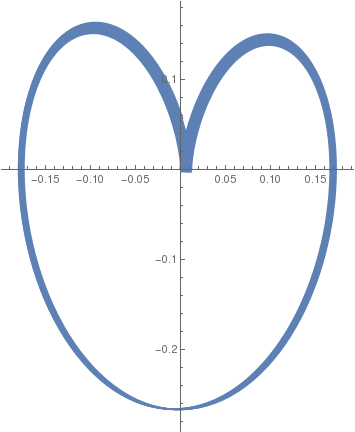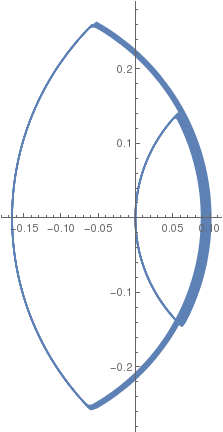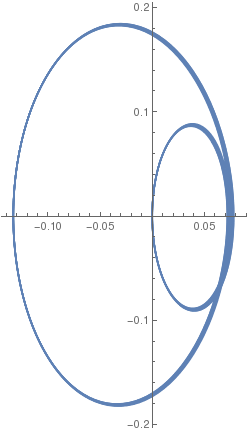F = (1/5) sign(sin(2.5 t)) F = (1/5) sin(2.5 t) F = (1/5) sign(cos(2.5 t)) F = (1/5) cos(2.5 t)
β = 1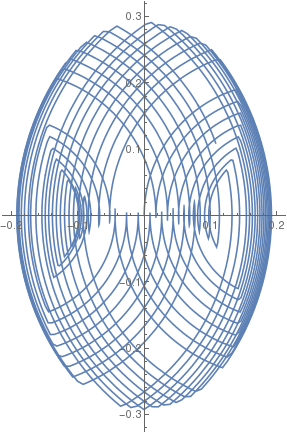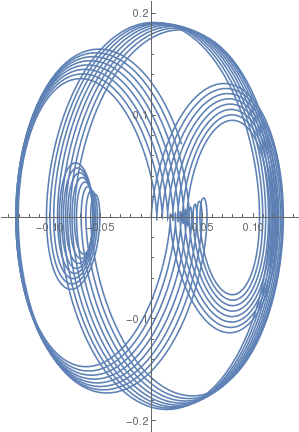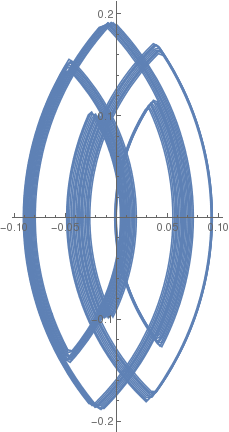β = 1/3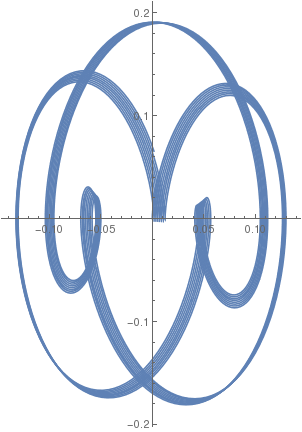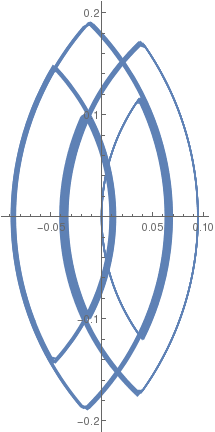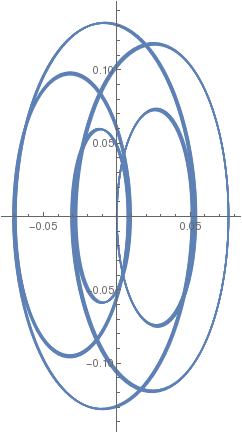β = 1/5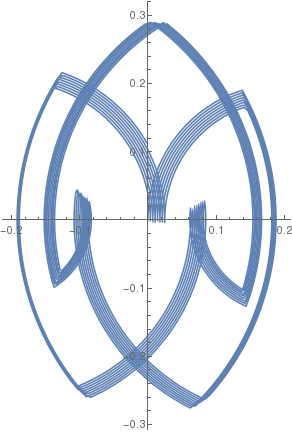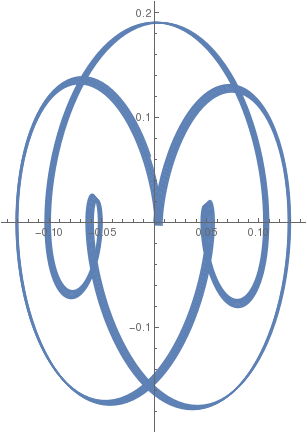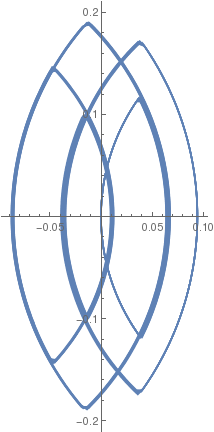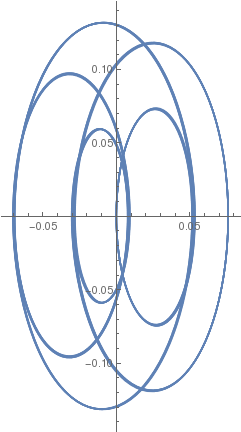β = 1/10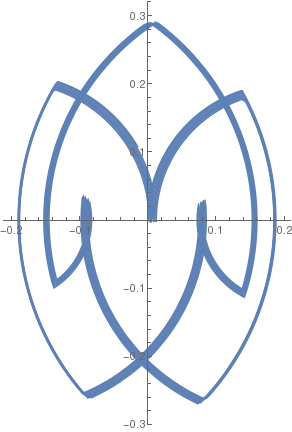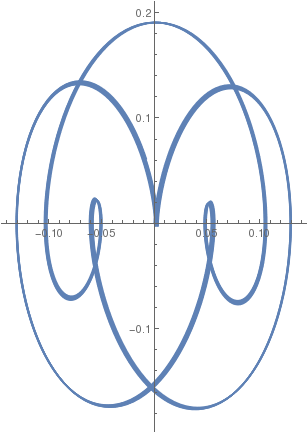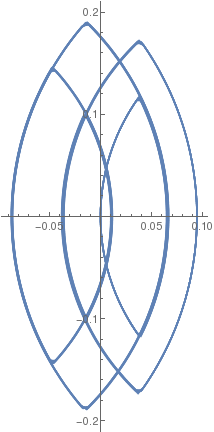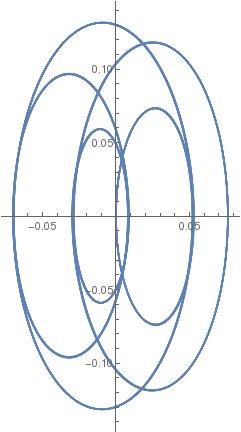Next, we increase the amplitude from 1/5 to 1/3:

F = (1/3) sign(sin(2.5 t)) F = (1/3) sin(2.5 t) F = (1/3) sign(cos(2.5 t)) F = (1/3) cos(2.5 t)
β = 1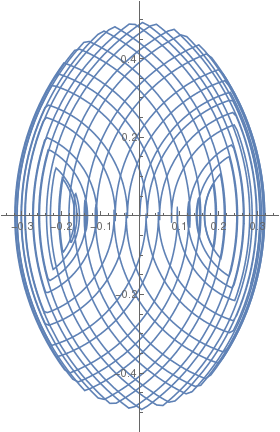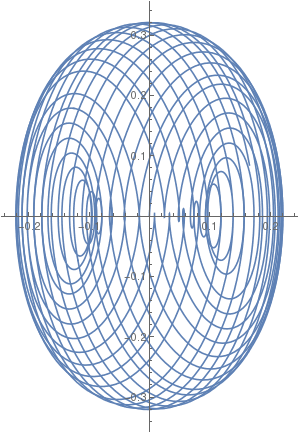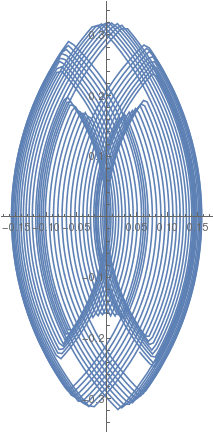β = 1/3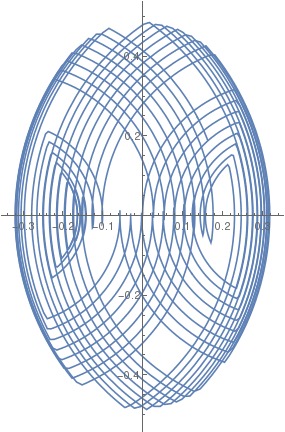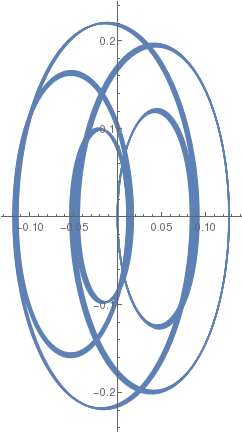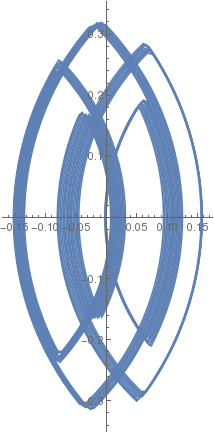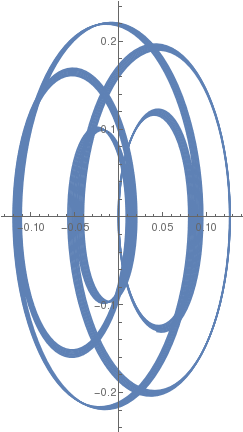β = 1/5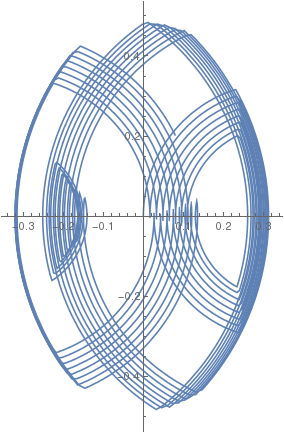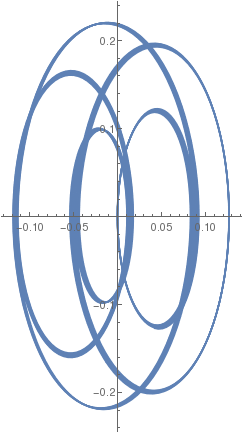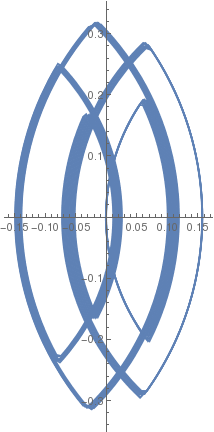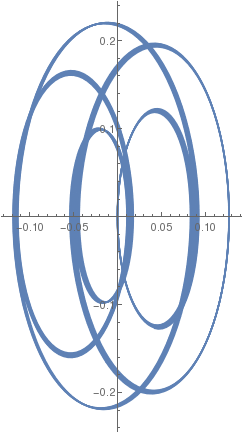β = 1/10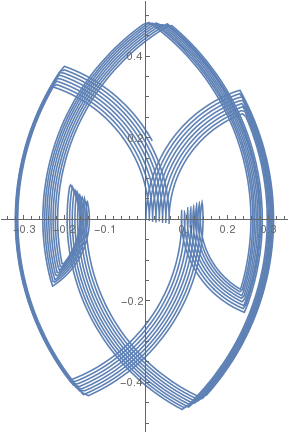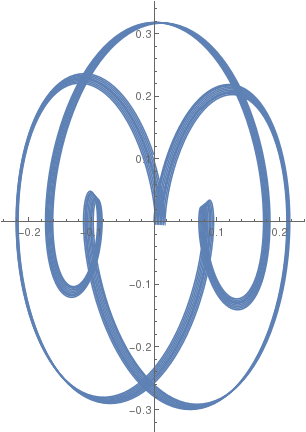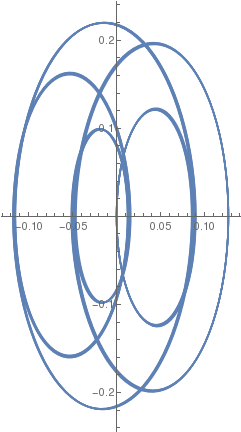# Frequency Response

The forced Duffing oscillator with cubic nonlinearity is described by the following ordinary differential equation:
$\ddot{x} + \delta \dot{x} + \alpha\,x + \beta\,x^3 = \Gamma \cos (\omega t) ,$
where the (unknown) function x = x(t) is the displacement at time t, {\dot {x}} is the first derivative of x with respect to time, i.e. velocity, and {\ddot {x}} is the second time-derivative of x, i.e. acceleration. The numbers δ , α, β , Γ and ω are given constants.

The frequency response of this oscillator describes the amplitude z of steady state response of the equation (i.e. x(t)) at a given frequency of excitation \omega . For a linear oscillator with \beta =0, the frequency response is also linear. However, for a nonzero cubic coefficient, the frequency response becomes nonlinear. Depending on the type of nonlinearity, the Duffing oscillator can show hardening, softening or mixed hardening–softening frequency response. Anyway, using the homotopy analysis method or harmonic balance, one can derive a frequency response equation in the following form:

# Damped Driven Hard Spring

1. Brennan, M.J.; Kovacic, I.; Carrella, A.; Waters, T.P., On the jump-up and jump-down frequencies of the Duffing oscillator, Journal of Sound and Vibration, 2008, Vol. 318, Issues 4–5, pp. 1250--1261. doi:10.1016/j.jsv.2008.04.032
2. Duffing, G., Erzwungene Schwingungen bei veränderlicher Eigenfrequenz und ihre technische Bedeutung [Forced oscillations with variable natural frequency and their technical relevance] (in German), 1918, Heft 41/42, pp. 134.
3. Fay, T.H., the forced hard spring equation, International Journal of Mathematical Education in Science and Technology, 2006, Vol. 37, No. 2, pp. 187--200; doi: 10.1080/00207390500285735
4. Jordan D.W. and Smith P., Nonlinear Ordinary Differential Equations An introduction for Scientists and Engineers, fourth edition, Oxford University Press, Oxford, 2007.
5. Liao S (2003) Beyond perturbation introduction to homotopy Analysis method. CRC Press
6. Liao S (2004a) On the homotopy analysis method for nonlinear problems. Appl Math Comput 147:499–513. doi: 10.1016/S0096-3003(02)00790-7
7. Liao S (2004b) An analytic approximate approach for free oscillations of self-excited systems. Int J Non Linear Mech 39:271–280
8. Liao S (2009) Notes on the homotopy analysis method: some definitions and theorems. Commun Nonlinear Sci Numer Simul 14:983–997. doi: 10.1016/j.cnsns.2008.04.013
9. Liao S (2012) Homotopy Analysis Method in Nonlinear Differential Equations. Springer
10. Kovacic, I.; Brennan, M.J., eds. (2011), The Duffing Equation: Nonlinear Oscillators and their Behaviour, Wiley, 392 pp., ISBN 978-0-470-71549-9
11. Tajaddodianfar, F.; Yazdi, M.R.H.; Pishkenari, H.N., Nonlinear dynamics of MEMS/NEMS resonators: analytical solution by the homotopy analysis method, Microsystem Technologies, 2016, doi:10.1007/s00542-016-2947-7
12. Duffing equation, WikiVisually